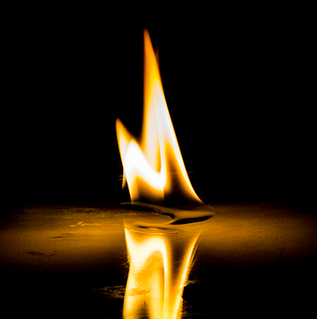Thermochemistry
4 years ago
mmassey
Save
Edit
Host a game
Live GameLive
Homework
Solo Practice
Practice15 QuestionsShow answers
• Question 1
30 seconds
Q. In an exothermic process the surrounding looses heat.
True
False
• Question 2
180 seconds
Q. In an endothermic reaction the system is releasing energy.
True
False
• Question 3
120 seconds
Q. Is the combustion of gasoline endothermic or exothermic?
Endothermic
Exothermic
• Question 4
120 seconds
Q. As someone is running on the track they begin to perspire.  If the runner is our system, are they endothermic or exothermic?
Endothermic process
Exothermic process
• Question 5
180 seconds
Q. A reaction is performed in a beaker with a temperature probe recording the temperature changes of the reaction.  If the temperature began at 15.0 degrees Celsius and ended at 27.5 degrees Celsius.  If the reaction is our system, is the system endothermic or exothermic?
Exothermic
Endothermic
• Question 6
300 seconds
Q. Refer the the following question:
2N2 + 3H2 --> 2NH3 + 46 kJ
How much energy would be produced if only 1 mol of nitrogen was reacted?
92 kJ
0.143 kJ
23 kJ
15 kJ
• Question 7
300 seconds
Q. How much energy must be used to produce 4.75 mol of gaseous water?
H2O (l) +  44.0 kJ --> H2O (g)
207 kJ
9.36 kJ
206.8 kJ
9.362 kJ
• Question 8
900 seconds
Q. What mass of P4 must be reacted to produce 5905 kJ of energy?
P4 + 6Cl2 --> 4PCl3 + 2439 kJ
25.43 g
563.0 g
2.421 g
300.0 g
• Question 9
120 seconds
Q. Do reactants in an endothermic reaction have a higher or lower energy than the products?
Higher
Lower
• Question 10
120 seconds
Q. Do the reactants in an exothermic reaction have a higher or lower energy than the products?
Higher
Lower
• Question 11
300 seconds
Q. 4 PCl5 + 3438 kJ --> P4 + 10 Cl2
How much energy is required to produce each mole of chlorine?
687.6 kJ
859.5 kJ
343.8 kJ
245.8 kJ
• Question 12
300 seconds
Q. In a laboratory experiment a student was using Styrofoam cups and foil to reduce the energy lost during an exothermic reaction.  The reaction constantly had a temperature probe in it.  Towards the end of the reaction the student noticed the temperature in the cups was going down (opposite what they thought is should).  The student concluded that the energy was destroyed within the system.  This student's reasoning violates what law?
Law of Conservation of Mass
Law of Enthalpy
First Law of Thermodynamics
Law of Heat Transfer
• Question 13
60 seconds
Q.

During an endothermic reaction in a beaker if we are part of the surroundings and touched the beaker, it would feel _______.

Warm

Cold

Bubbly

Damp

• Question 14
60 seconds
Q. H2 + Cl2 --> 2 HCl + 1845 kJ
Is this reaction endothermic or exothermic?